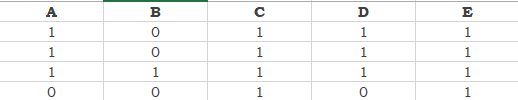# Column expression formula

I don’t know how to program in column expression I’m trying to learn, but I can’t find an exercise like the one I need

I have 5 columnsThe formula I use to do this in EXCEL is relatively simple
=+SI(Y(A=1;B=0);1;0)
and I drag this for all the cells, I have not found a way to program this

I did a horrible thing hahaha but it worked for me however I know it’s not the best way

An expression for each operation
then I counted only one

I would really like to receive suggestions for not doing these ugly things

Your 100% above my Excel SkillsWhat does this formula do?

Did you check the Math Formula (Multi Column) ?

Hi @Jalvear
I would suggest a ‘Rule Engine’ node:

Columns as strings:

``````\$A\$ LIKE "1" AND \$B\$ LIKE "0" => 1
TRUE => 0
``````

Columns as numeric:

``````\$A\$ = 1 AND \$B\$ = 0 => 1
TRUE => 0
``````

BR

This is a solution but for only one column I need the following rule to be
\$B\$ LIKE “1” AND \$C\$ LIKE “0” => 1
TRUE => 0
and so with the 50 columns that I have, but I don’t see it appropriate to create a 50 rule engine, so let’s say that I was looking for a way to simulate this excel function in knime for multiple columns, since in excel it is relatively easy to drag the function horizontally and vertically

In general terms, if the previous month was 1 and the current month is 0, place 1, otherwise place 0.

1 Like

You can always build your logic and feed input outputs as variables in a looped workflow.

Otherways moving into scripting is an option.

BR

This topic was automatically closed 90 days after the last reply. New replies are no longer allowed.